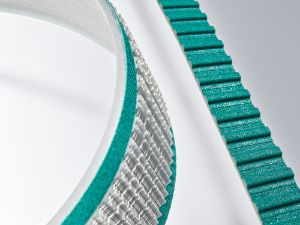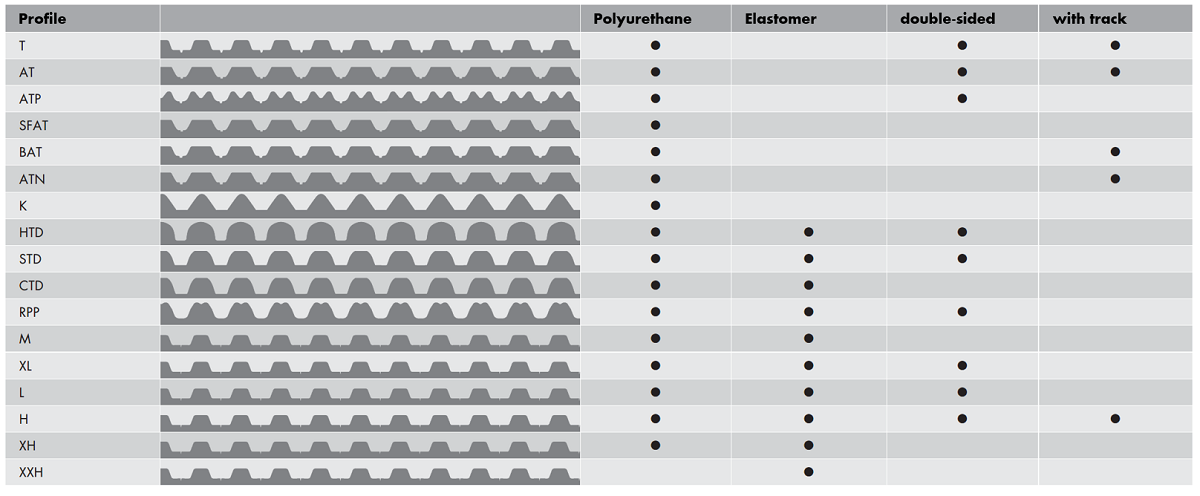# 线性和运输技术用 APSOdrive® 皮带

## 高品质的同步带和自定义表面处理

••• Low maintenance applications
• Low noise applications
• High shock applications
• High vibration applications
• Low friction applications

APSOdrive® 齿型可用于无缝闭口带与开口带、皮带轮和夹板• 横向和纵向打磨皮带
• 背面和皮带边缘磨削
• 标准穿孔和水切割
•  涂层（> 50 种标准涂料和 > 20 种客户特定涂料）
•  喷涂或硫化涂层
• 挡块和刷体焊接、粘合，在皮带背面拧上锥/钉/针/鬃毛
• 3000 多种标准挡块
• 金属嵌件、磁性嵌件

## 产品表

       BRECOflex® 完全闭口型同步带 聚氨酯 产品名称 产品说明 材料 初选 后期处理能力 优势 重要应用领域 订购 标准节距，单侧 英制节距 自导式 带轨道的自导式 齿侧尼龙面 背侧尼龙面 机械加工 焊接夹板和型材 涂布 提高动力传输率 精准定位 用于无菌环境 耐油、耐脂 高环境温度（高于 +80°C） 低维护应用 低噪音应用 低摩擦应用 高冲击应用 高振动应用 x x x x x x x x x x x x x x x x x BRECO-V® 焊接型同步带 聚氨酯 x x x x x x x x x x x x x x x BRECO-M® 开口同步带 聚氨酯 x x x x x x x x x x x x x x x x x BRECO-ATN® 弯曲型同步带系统 聚氨酯 x x x x x x x x x x BRECOprotect® 食品和医疗加工同步带 聚氨酯 x (x) x x (x) (x) (x) x x x x x x x BRECOmove® 高性能同步带 聚氨酯 x x x x x x x x x x BRECOclassic® 替代标准型同步带 聚氨酯 x x x x x x x x x x (x) (x) x x x BRECObasic® 经济标准型同步带 聚氨酯 x (x) x (x) (x) Continental Synchrodrive® 开口同步带 聚氨酯 x x x x (x) x x x x x 平皮带 适于提升系统中空间节约型传动配置的平皮带 聚氨酯/橡胶 (x) x x x x x x x x x x 传输带 适合物料运输和操作处置的专用平皮带 聚氨酯/橡胶 (x) x x (x) x x (x) (x) x x x x 模块化皮带 智能模块化输送带 聚氨酯 (x) x (x) (x) x x 圆带 圆形截面带 聚氨酯/橡胶 x x x x x x (x) (x) (x)

### ATN 同步带

ATN 同步带专为运输技术的应用而设计。皮带牙齿中的可更换紧固系统可供快速安装挡块，且可针对特定输送应用单独定制。与其他焊接的紧固系统相比，这种灵活性实现了前所未有的各种可能性。通过 ATN 同步带，可以在使用相同同步带、但配备不同挡块装置的一个运输系统中，输送不同类型的货物。

### BRECOprotect® 同步带

BRECOprotect® 产品线专为食品和制药行业而设计。BRECOprotect® 皮带里面的钢丝完全封闭于聚氨酯中以防腐蚀。根据食品和制药行业的要求，所有 BRECOprotect® 同步带的聚氨酯均符合 FDA 177.1680、欧洲 1935/2004 法规、欧盟 10/2011、欧盟委员会 90/128/CEE 和 96/11/CE 指令的标准。该产品是蓝色系列,紧跟市场大势，是采用一种特殊的食品行业配色方案。

### BRECOclassic® 同步带

BRECOclassic® 同步带由高成本效益的白色聚氨酯制成，其邵氏硬度为 92A (TPUCLA)，并配备专为适应 BRECOclassic® 而优化设计的线芯。